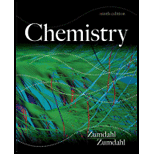# What is a half-reaction? Why must the number of electrons lost in the oxidation half reaction equal the number of electrons gained in the reduction half-reaction? Summarize briefly the steps in the hall-reaction method for balancing redox reactions. What two items must be balanced in a redox reaction (or any reaction)?### Chemistry

9th Edition
Steven S. Zumdahl
Publisher: Cengage Learning
ISBN: 9781133611097### Chemistry

9th Edition
Steven S. Zumdahl
Publisher: Cengage Learning
ISBN: 9781133611097

#### Solutions

Chapter
Section
Chapter 18, Problem 1RQ
Textbook Problem

## What is a half-reaction? Why must the number of electrons lost in the oxidation half reaction equal the number of electrons gained in the reduction half-reaction? Summarize briefly the steps in the hall-reaction method for balancing redox reactions. What two items must be balanced in a redox reaction (or any reaction)?

Expert Solution
Interpretation Introduction

Interpretation:

The definition for half reaction and balancing of a redox reaction using half-reaction method is to be stated.

Concept introduction:

A redox reaction consists of two parts, one is oxidation and the other is reduction. These two reactions are called half reactions and they are involved in the change of oxidation number of the atoms present in the reaction.

To determine: The definition for half reaction and the steps involved in the balancing of a redox reaction using the half-reaction method; the reason behind the loss and gain of equal number of electrons in the oxidation and reduction half-reaction respectively; the steps involved in the half-reaction method for balancing the redox reaction and the two items that must be balanced in a redox reaction (or any chemical reaction).

A half reaction is that type of reaction that involves either oxidation or reduction of a redox reaction.

It occurs because of the change in the oxidation number of the atoms involved in the reaction.

The number of electrons lost in the oxidation half-reaction must be equal to the number of electrons gained in the reduction half-reaction, to balance the charge of the overall reaction.

The number of electrons and atoms must be balanced in a redox reaction (or any reaction)

### Explanation of Solution

The half reaction consists of either oxidation half or reduction half of a redox reaction.

The reaction in which transfer of electrons occur from one species to another is called redox reaction. The species which lose electron is said to be oxidized while the species which gain electron is reduced.

So, a redox reaction consists of two reactions, one is the oxidation half and the other is reduction half.

A half reaction can be either oxidation one or reduction one and it occurs because of the change on the oxidation number (state) of the species involved in the reaction.

The number of electrons lost during oxidation and those gained during reduction must be equal to maintain the overall charge of the redox reaction.

The equal gain and loss of electrons can be explained as follows,

Cu(s)Cu2+(aq)+2e (1)

2Ag+(aq)+2e2Ag(s) (2)

Cu(s)+2Ag+(aq)Cu2+(aq)+2Ag(s) (3)

To balance the overall charge and mass, the equation (2) is multiplied by 2 that gives the final equation (3) that satisfies both the required conditions.

The steps involved in the balancing of redox reaction by half-reaction method can be summarized as follows.

In acidic medium, the reaction of reaction of Fe(II) with potassium permanganate is,

FeSO4+KMnO4+5H2SO4Fe2(SO4)3+MnSO4+H2O+K2SO4 (4)

The ionic reaction can be represented as,

Fe2++MnO4+H+Fe3++Mn2++H2O (5)

• The oxidation half and reduction half of the reaction, respectively, is,

Fe2+Fe3+ (6)

MnO4Mn2+ (7)

• Balance the oxidation half-reaction by the addition of one electron to the right hand side of the reaction.

Fe2+Fe3++e (8)

• Balance the reduction half-reaction. As the oxygen atoms are not balanced, try to balance them by adding equal number of water molecules on the opposite side.

MnO4Mn2++4H2O (9)

• Balance the hydrogen atoms by adding equal number of H+ on the opposite side.

MnO4+8H+Mn2++4H2O (10)

• The charge on Mn at left side is +7 while on right side is +5 Balance the charge on both sides by adding 5 electrons on the left hand side.

MnO4+8H++5eMn2++4H2O (11)

• To balance the overall charge, multiply equation (8) by 5.

5Fe2+5Fe3++5e (12)

• Add equation (11) and (12).

MnO4+8H++5Fe2++5eMn2++4H2O+5Fe3++5e- (13)

• To write the final equation, cancel out the electrons on both the sides.

MnO4+8H++5Fe2+Mn2++4H2O+5Fe3+ (14)

The number of atoms and electrons must be balanced in a redox reaction (or any chemical reaction).

According to the law of conservation of mass, mass can neither be created nor destroyed in a chemical reaction.  Therefore mass of every species must be conserved in the reaction.  Therefore to balance mass, the number of atoms must be balanced in a chemical equation.  Besides this the charge on both the sides of the reaction must be balanced to maintain the overall charge of the reaction.

Conclusion

The redox reaction consists of the reduction half-reaction and oxidation half-reaction. The reaction must be properly balanced to maintain the law of conservation of mass and overall charge.

### Want to see more full solutions like this?

Subscribe now to access step-by-step solutions to millions of textbook problems written by subject matter experts!

Get Solutions

### Want to see more full solutions like this?

Subscribe now to access step-by-step solutions to millions of textbook problems written by subject matter experts!

Get Solutions